# Take the breakdown voltage of the zener diode used in the given circuit as 6V.Question:

Take the breakdown voltage of the zener diode used in the given circuit as 6V. For the input voltage shown in figure below, the time variation of the output voltage is :

(Graphs drawn are schematic and not to scale)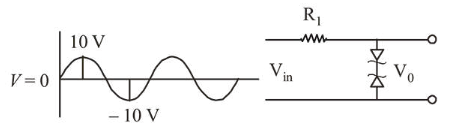1.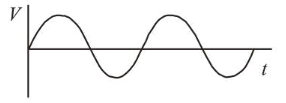2.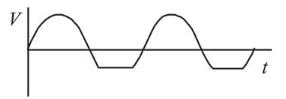3.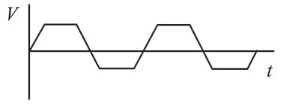4.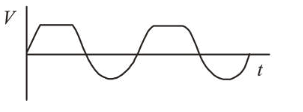Correct Option: , 3

Solution:

(3) Here two zener diodes are in reverse polarity so if one is in forward bias the other will be in reverse bias and above $6 \mathrm{~V}$ the reverse bias will too be in conduction mode. Hence when $V>6 V$ the output will be constant. And when $\mathrm{V}<6 \mathrm{~V}$ it will follow the inut voltage.